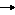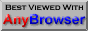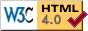## Chapter 9 - Conditional Execution

In the cases we have seen so far all the code is executed when the program is run. Many times this is not what is required. There needs to be a way of running a block of code only if certain conditions are met. This need is so fundamental to programming languages that all have it and many (PASCAL, C/C++, JAVA and, of course, BASIC to name some) use the same word: IF.

Example:

 INPUT "Enter your score: " Score% IF Score%>40 THEN PRINT "You passed!" END
Line 2 makes a test on the variable Score% and the PRINT statement is only run if the condition is found to be true. A full list of comparisons is given below, but as can be seen common sense and basic maths were used in their implementation.

Expression Evaluates to true when
A <  B A is less than B
A <= B A is less than or equal to B
A =  B  A is equal to B
A >= B  A is greater than or equal to B
A >  B  A is greater than B
A <> B A is not equal to B

We can expand our age test program:
 INPUT "Enter your score: " Score% IF Score%>40 THEN PRINT "You passed!" IF Score%=40 THEN PRINT "You just passed!" IF Score%<40 THEN PRINT "You failed!" END
Furthermore, A and B can be expressions in themselves:
 IF MyAge%=YourAge%+5 THEN PRINT "I'm older"
When used in an expression like the above, = acts as a comparison not an assignment, so no values would actually be altered during this test.

It is perfectly possible to test strings as well. In this case, the test is made on the value of the characters from the ASCII table, as mentioned in the chapter on strings. When comparing strings, BASIC will start with the first character of the first string and compare it with the first character of the second string. If they are the same, it will test the second characters of both strings and so on, until it can make a decision. Here are some examples:

 IF "abc"="abc" PRINT "both the same" IF "abc"<"bcde" PRINT "a comes before b" IF "abc"<"abde" PRINT "c comes before d" IF "abc"<>"ABC" PRINT "different cases" IF "ABC"<"abc" PRINT "upper case is first" END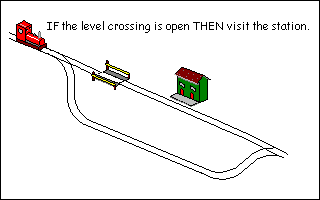AND and OR

Type in and run this little program:

 REM Quiz INPUT "Is London the capital of England";A\$ IF A\$="Y" THEN PRINT "Correct" IF A\$="N" THEN PRINT "Wrong" END
Line 3 above would only work if the user typed an uppercase 'Y'. If they typed  'y', they would never find out how clever they are. Not very friendly. It is possible to combine more than one condition at a time using keywords AND and OR.  We can modify lines 3 and 4 to do this:
 REM Quiz INPUT "Is London the capital of England";A\$ IF A\$="Y" OR A\$="y" THEN PRINT "Correct" IF A\$="N" OR A\$="n" THEN PRINT "Wrong" END
This is much more usable.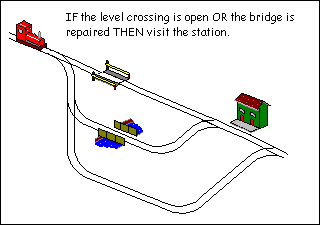We can also add a trap for an incorrect response:

 REM Quiz INPUT "Is London the capital of England";A\$ IF A\$="Y" OR A\$="y" THEN PRINT "Correct" IF A\$="N" OR A\$="n" THEN PRINT "Wrong" IF A\$<>"Y" AND A\$<>"y" \ \ AND A\$<>"N" AND A\$<>"n" \ \ THEN PRINT "Invalid response" END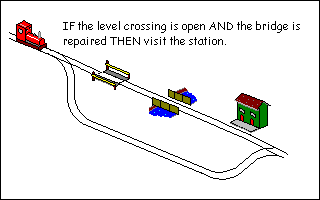It is customary at this point to provide truth tables of the various conditions:

 AND When A is AND B is Result False False False True False False False True False True True True

 OR When A is OR B is Result False False False True False True False True True True True True

Reading the third line of the AND table tells us if A is false and B is true, the overall result will be judged as false. Common sense really.

EOR

EOR (which to the delight of students everywhere is pronounced like the donkey in Winnie the Pooh) stands for Exclusive OR. It will test for a condition like OR but will only be true when one test is true, not both. Perhaps a truth table will explain it better.

 EOR When A is EOR B is Result False False False True False True False True True True True False

NOT

NOT is another word that can be used in comparisons. Unlike AND, OR and EOR, it is not used to join statements but simply negates a condition. As an example, take the test for a negative response at the end of our quiz program:

 REM ... IF A\$="Y" OR A\$="y" THEN PRINT "Correct" IF A\$="N" OR A\$="n" THEN PRINT "Wrong" IF A\$<>"Y" AND A\$<>"y" \ \ AND A\$<>"N" AND A\$<>"n" \ \ THEN PRINT "Invalid response" END
As line 4 is a bit long, we decide that any response which is not "Y" or "y" can produce the same message. Line 3 can be rewritten and line 4 removed:
 IF NOT(A\$="Y" OR A\$="y") THEN PRINT "Wrong" END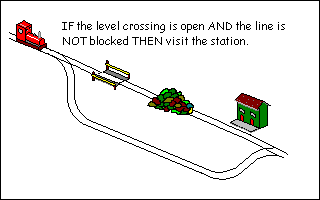There are a large variety of words that can be used here. Don't let them confuse you, they are there to be used to make your program more intelligible. If you find your logic tests are tying your head in knots (or NOTs, groan), there's probably a better way to express it, try restructuring.

ELSE

In this example, we want to set a variable to say whether the user passed or failed a test. The variable could be used in IF statements throughout the rest of the program. We could do this:

 INPUT "Enter your score: " Score% IF Score%>=40 THEN Pass=1 IF Score%<40 THEN Pass=0 END
However, it does seem a bit inefficient using two mutually exclusive lines. In this case, IF can be combined with ELSE.
 INPUT "Enter your score: " Score% IF Score%>=40 THEN Pass=1 ELSE Pass=0 END
If Score%>=40, the first assignment is executed. If it's false, the second assignment is executed. It's not too different from English really and if the pass mark changes, there's only one line to alter.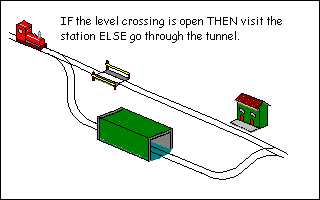The word THEN is usually optional on single line IF statements (multi-line ones are coming up next) provided the meaning is clear:

 IF Score%>=40 Pass=1 ELSE Pass=0
Multi-line IF statements

BB4W allows you to split the IF statement across multiple lines. For example, it's possible to do something like this:

If Salary is more than 1 million (assuming we're not in Turkey here!) then both the variables will be set to 1. If we were really affluent, we might also want to buy a helicopter as well. Adding to our line puts us in danger of unreadable lines that scroll off the edge of the screen. Isn't it much easier to read it like this:
That way we can run lots of code conditionally. When THEN is the last statement on a line (not even a REM is allowed here), BB4W knows that it is about to be presented with a multi-line IF. The end of the block is denoted by the corresponding ENDIF statement. Never put a space in ENDIF. Some other dialects of BASIC will accept END IF as two separate words. BBC BASIC won't. It will take the first END to mean the end of the program and stop dead. The editor will automatically indent blocks like this so it is easier to read. If the indentation goes awry, there's a warning that something is wrong.

You can also use ELSE in this structure as well:

Each IF statement can only have one corresponding ENDIF and, optionally, one ELSE. It is possible to nest statements:
 IF Salary>1000000 THEN BuyYacht=1 IF ReallyExtravagant=1 THEN BuyVilla=1 BuyHelicopter=1 ENDIF ELSE PRINT "Work, work and more work" ENDIF
Operator precedence

It is possible to combine comparisons with AND and OR statements in any combinations that logic allows, but be careful because BASIC's interpretation might not be yours.

 IF Raining=1 OR Snowing=1 AND Boots=0 THEN PRINT "You'll need your boots" ENDIF
If we go back to our table of operator precedences in Chapter 5, we can see that AND has priority over OR. The line will be interpreted like this:
 IF Raining=1 OR (Snowing=1 AND Boots=0) THEN PRINT "You'll need your boots" ENDIF
If it's raining, you'll always be told to take your boots, whether you have them or not. Only if it's snowing and you've no boots will you be prompted for them. When in doubt, use parentheses:
 IF (Raining=1 OR Snowing=1) AND Boots=0 THEN PRINT "You'll need your boots" ENDIF
TRUE and FALSE

As an end to this section, I would like to mention that BBC BASIC has two predefined variables: TRUE and FALSE. These actually have values, to see them go into immediate mode and type:

 PRINT TRUE, FALSE
This might not seem like a particularly useful thing to have, but it is great for testing yes / no situations and making a program more readable.
 REM Using TRUE / FALSE INPUT "Enter password " Password\$ IF Password\$="Super" THEN Supervisor%=TRUE ELSE Supervisor%=FALSE ENDIF IF Supervisor% THEN REM Show main configuration screen PRINT "Welcome, master" ELSE REM Show ordinary user's screen PRINT "What do you want now?" ENDIF END
The variable used to test true or false situations is often referred to as a flag. If this program was a lot bigger we could use the flag repeatedly instead of making the same comparison. That way, when the password changes, we only have to change one line of code, rather than lots. Look at line 8. This is equivalent to IF Supervisor%=TRUE ... but BASIC is clever enough to insert the implied =TRUE for us when it runs, again making the code more readable. If you wanted to test the reverse condition, you would still need to put IF Supervisor%=FALSE ... or IF NOT Supervisor% ...

Tip: Disabling a block of code
Any IF expression is evaluated until it returns TRUE or FALSE. To disable code whilst testing, we can wrap it in block, like this:
 REM Some code IF FALSE THEN CLS PRINT "Introductory screens" ENDIF REM Rest of code
IF looks for the condition to be true. FALSE, by definition, is never true, therefore the code will never be run.

Exercise

Below is a sample output from two runs of a program that acts as a simple calculator:

 Enter first number: 5 Enter second number: 6 Enter 1 to add or 2 to subtract: 1 5 + 6 = 11 >RUN Enter first number: 45 Enter second number: 55 Enter 1 to add or 2 to subtract: 2 45 - 55 = -10 >
Can you write the program that produces the above screen? Allow one INPUT for each number, one INPUT for the operations. Use IF to select the correct PRINT statement to display the result. Extra marks if you expand to include multiply and divide.

### CHAPTER 10